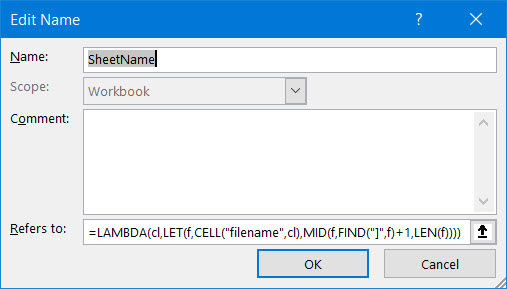2023
Happy new year to you and your loved ones!

## The LAMBDA function

If you have a Microsoft 365 subscription you may have heard about the new LAMBDA function and wondered "What kind of strange function is that?".

In short: with this new LAMBDA function you can create your own worksheet functions, without writing a single line of (VBA) code.

### An example

A request I've heard a lot in the many years answering forum questions is this one: I need an Excel formula that will give me the name of the worksheet tab in any cell of that worksheet.

This is a simple enough request, with a relatively complicated answer:

=MID(CELL("filename",A1),FIND("]",CELL("filename",A1))+1,LEN(CELL("filename",A1)))

Not something I'd like to type each time. I'd prefer writing: =SheetName(A1)

We can use the LET function to make the aforementioned formula slightly simpler:

=LET(f,CELL("filename",A1),MID(f,FIND("]",f)+1,LEN(f))

Now we're just two steps away from transforming this into a Lambda.

1. Add the LAMBDA function to the previous formula (the only argument is a cell on the worksheet from which you want to display the name):

=LAMBDA(cl,LET(f,CELL("filename",cl),MID(f,FIND("]",f)+1,LEN(f)))

2. Define a name called SheetName and paste this formula into the Refers To box:That's it, you can now get the sheet name into a cell with this simple formula:

=SheetName(A1)

 Copyright © 2023 JKP Application Development Services, All rights reserved. unsubscribe from this list    update subscription preferences